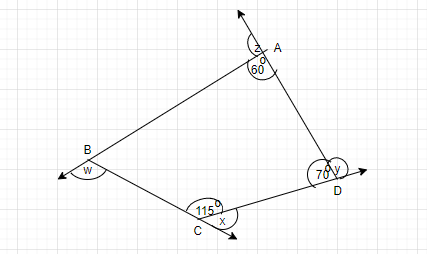QUESTION

# Find x + y + z + w in the given figure.Hint: Here we will proceed by using the sum of interior angle properties of the quadrilateral i.e. ${360^ \circ }$because the given shape is quadrilateral. Also we will use exterior angle property.

Quadrilateral is a polygon with four edges and four vertices or corners.
We will use the property of the quadrilateral that sum of all the interior angles is ${360^ \circ }$
$\Rightarrow$ ${115^ \circ }$ + ${60^ \circ }$ + ${70^ \circ }$ = ${245^ \circ }$
$\Rightarrow$ ${245^ \circ }$+ $\angle ABC$= ${360^ \circ }$
$\Rightarrow $\angle ABC = {360^ \circ }- {245^ \circ } \Rightarrow$\angle ABC$ = ${115^ \circ }$
Now using the exterior angle property,
We get x = exterior angle $\angle BCD$
$\Rightarrow$x =${180^ \circ }$- $\angle BCD$
$\Rightarrow$x =${180^ \circ }$- ${115^ \circ }$= ${65^ \circ }$
Similarly, y = ${180^ \circ }$- ${70^ \circ }$= ${110^ \circ }$
$\Rightarrow$ z = ${180^ \circ }$- ${60^ \circ }$= ${120^ \circ }$
$\Rightarrow$w = ${180^ \circ }$- ${115^ \circ }$= ${65^ \circ }$
Now add all the calculated values of x, y, z, w
$\therefore$ x + y + z + w =${65^ \circ }$+ ${110^ \circ }$+ ${120^ \circ }$+ ${65^ \circ }$
$\Rightarrow$${360^ \circ }$

Note: We can solve this type of question by other ways like linear pair axioms. Also we must know the properties of quadrilaterals such as interior angle property and exterior angle property. Hence we will get the desired result.